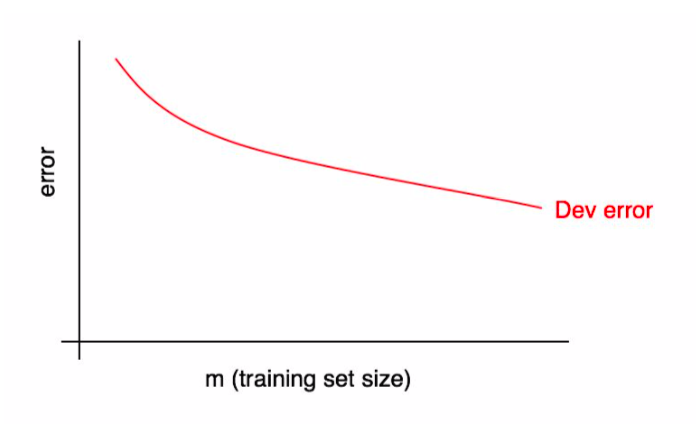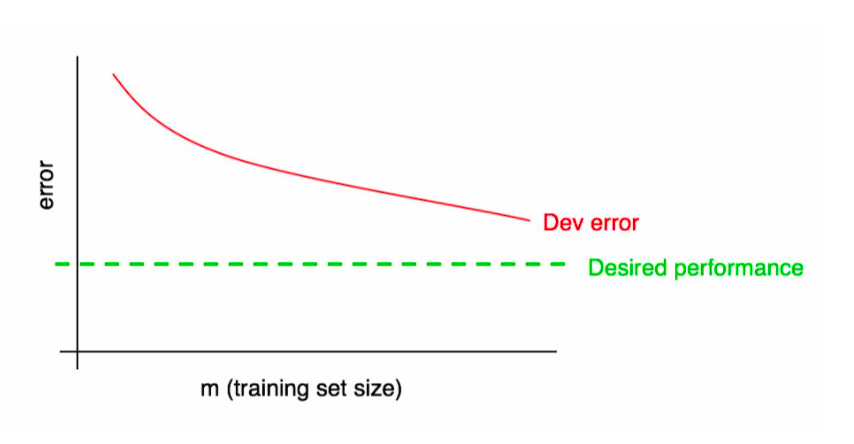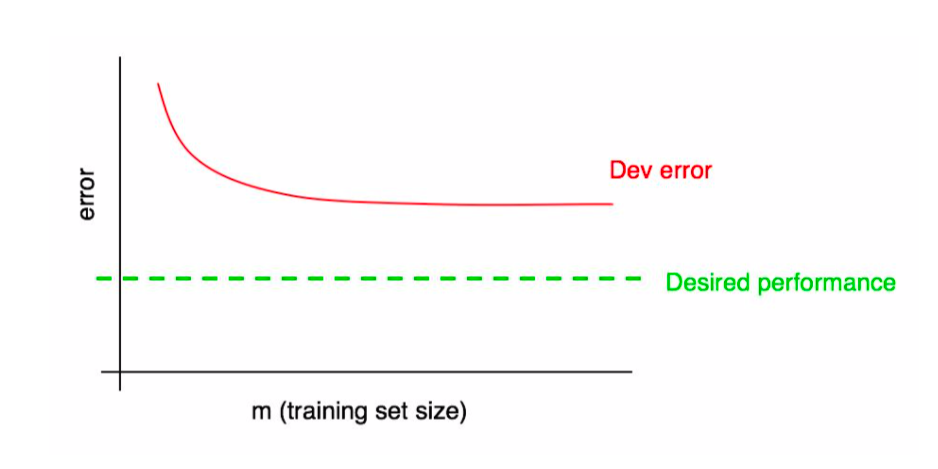# Lecture 22: Tools for Diagnosing Model Performance¶

### Applied Machine Learning¶

Volodymyr Kuleshov
Cornell Tech

# Practical Considerations When Applying Machine Learning¶

Suppose you trained an image classifier with 80% accuracy. What's next?

• Train the algorithm for longer?
• Use a bigger model?

We will next learn how to prioritize these decisions when applying ML.

# Part 1: Learning Curves¶

Learning curves are a common and useful tool for performing bias/variance analysis in a deeper way.

This section is mostly based on materials from an e-book by Andrew Ng.

# Review: Overfitting (Variance)¶

Overfitting is one of the most common failure modes of machine learning.

• A very expressive model (a high degree polynomial) fits the training dataset perfectly.
• The model also makes wildly incorrect prediction outside this dataset, and doesn't generalize.

Models that overfit are said to be high variance.

# Review: Underfitting (Bias)¶

Underfitting is another common problem in machine learning.

• The model is too simple to fit the data well (e.g., approximating a high degree polynomial with linear regression).
• As a result, the model is not accurate on training data and is not accurate on new data.

Because the model cannot fit the data, we say it's high bias.

# Learning Curves¶

Learning curves show performance as a function of training set size.Learning curves are defined for fixed hyperparameters. Observe that dev set error decreases as we give the model more data.

# Visualizing Ideal Performance¶

It is often very useful to have a target upper bound on performance (e.g., human accuracy); it can also be visualized on the learning curve.Extrapolating the red curve suggests how much additional data we need.

In the example below, the dev error has plateaued and we know that adding more data will not be useful.# Learning Curves for the Training Set¶

We can further augment this plot with training set performance.